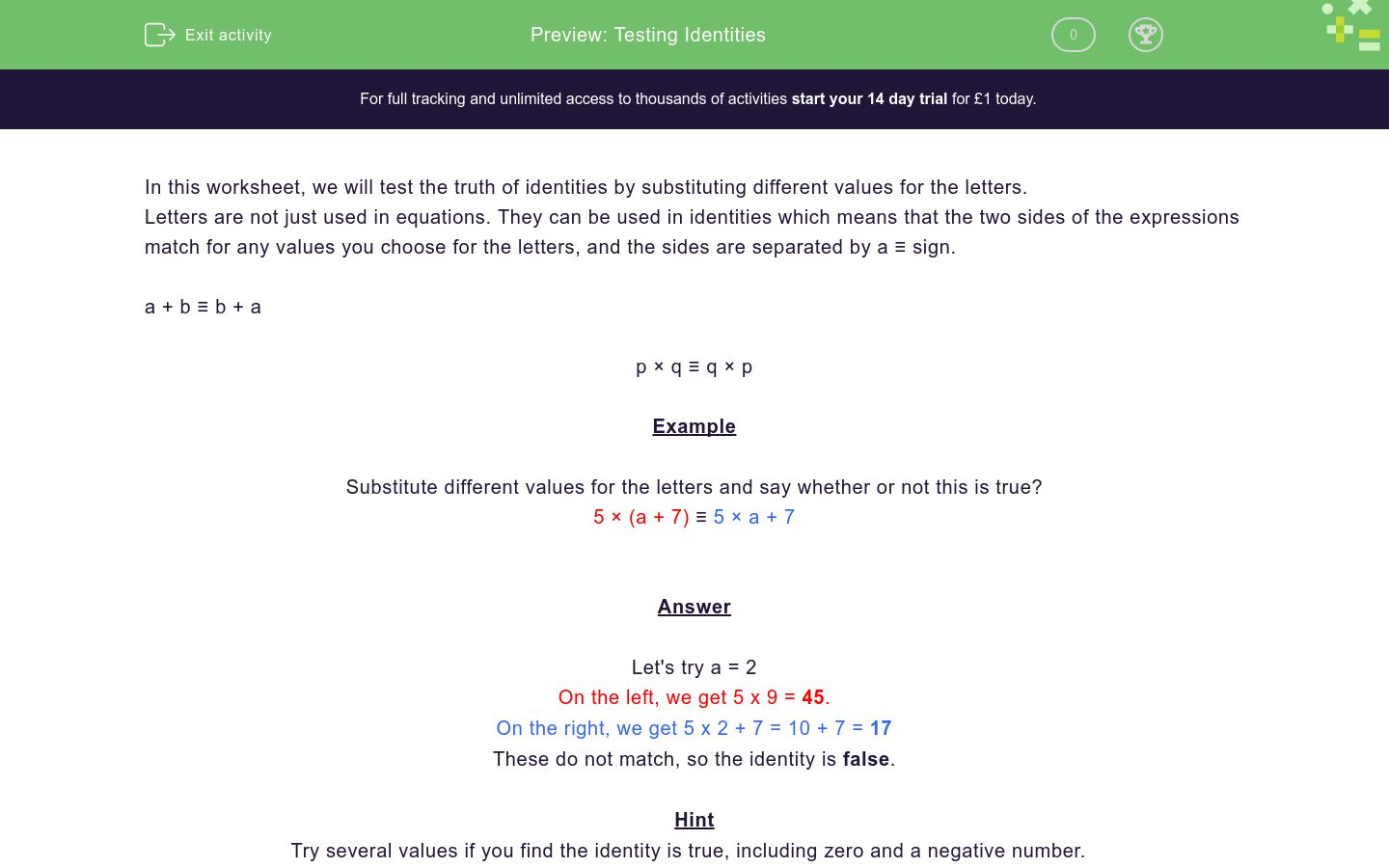# Testing Identities

In this worksheet, students test the validity of the given identities.Key stage:  KS 3

Curriculum topic:   Algebra

Curriculum subtopic:   Understand Expressions, Equations, Inequalities, Terms and Factors

Difficulty level:### QUESTION 1 of 10

In this worksheet, we will test the truth of identities by substituting different values for the letters.

Letters are not just used in equations. They can be used in identities which means that the two sides of the expressions match for any values you choose for the letters, and the sides are separated by a ≡ sign.

a + b ≡ b + a

p × q ≡ q × p

Example

Substitute different values for the letters and say whether or not this is true?

5 × (a + 7)5 × a + 7

Let's try a = 2

On the left, we get 5 x 9 = 45.

On the right, we get 5 x 2 + 7 = 10 + 7 = 17

These do not match, so the identity is false.

Hint

Try several values if you find the identity is true, including zero and a negative number.

Just to be sure!

Substitute different values for the letters and say whether or not this is true:

4 × x ≡ x + x + x + x + x

Yes

No

Substitute different values for the letters and say whether or not this is true:

a + b + c ≡ c − b + a

Yes

No

Substitute different values for the letters and say whether or not this is true:

5 × (a + 7) ≡ 5 × a + 7

Yes

No

Substitute different values for the letters and say whether or not this is true:

3 × (a + b) ≡ 3 × a + 3 × b

Yes

No

Substitute different values for the letters and say whether or not this is true:

a + b + c ≡ c + b + a

Yes

No

Substitute different values for the letters and say whether or not this is true:

5(a + 7) ≡ 5a + 35

Yes

No

Substitute different values for the letters and say whether or not this is true:

4 × n ≡ n + n + n + n

Yes

No

Substitute different values for the letters and say whether or not this is true:

14 + a ≡ a + 14

Yes

No

Substitute different values for the letters and say whether or not this is true:

a - 14 ≡ 14 - a

Yes

No

• Question 1

Substitute different values for the letters and say whether or not this is true:

4 × x ≡ x + x + x + x + x

No
EDDIE SAYS
To prove an inequality is wrong, we only have to use one number. If this is incorrect, it cannot be an identity. If we use x = 2, this gives us 8 = 10 which is incorrect.
• Question 2

Substitute different values for the letters and say whether or not this is true:

a + b + c ≡ c − b + a

No
EDDIE SAYS
To prove an inequality is wrong, we only have to use one number. If this is incorrect, it cannot be an identity. If we use a = 1, b = 2 and c = 3, this gives us 6 = 4 which is incorrect.
• Question 3

Substitute different values for the letters and say whether or not this is true:

5 × (a + 7) ≡ 5 × a + 7

Yes
EDDIE SAYS
This will work for any value of a
• Question 4

Substitute different values for the letters and say whether or not this is true:

3 × (a + b) ≡ 3 × a + 3 × b

Yes
EDDIE SAYS
This will work for any value of a and b
• Question 5

Substitute different values for the letters and say whether or not this is true:

a + b + c ≡ c + b + a

Yes
EDDIE SAYS
This will work for any values of a, b and c
• Question 6

Substitute different values for the letters and say whether or not this is true:

5(a + 7) ≡ 5a + 35

Yes
EDDIE SAYS
This will work for any value of a
• Question 7

Substitute different values for the letters and say whether or not this is true:

4 × n ≡ n + n + n + n

Yes
EDDIE SAYS
This will work for any value of n
• Question 8

Substitute different values for the letters and say whether or not this is true:

14 + a ≡ a + 14

Yes
EDDIE SAYS
This will work for any value of a
• Question 9

Substitute different values for the letters and say whether or not this is true:

a - 14 ≡ 14 - a

No
EDDIE SAYS
To prove an inequality is wrong, we only have to use one number. If this is incorrect, it cannot be an identity. If we use a=2, this gives us -12 = 12 which is incorrect.
---- OR ----

Sign up for a £1 trial so you can track and measure your child's progress on this activity.

### What is EdPlace?

We're your National Curriculum aligned online education content provider helping each child succeed in English, maths and science from year 1 to GCSE. With an EdPlace account you’ll be able to track and measure progress, helping each child achieve their best. We build confidence and attainment by personalising each child’s learning at a level that suits them.

Get started